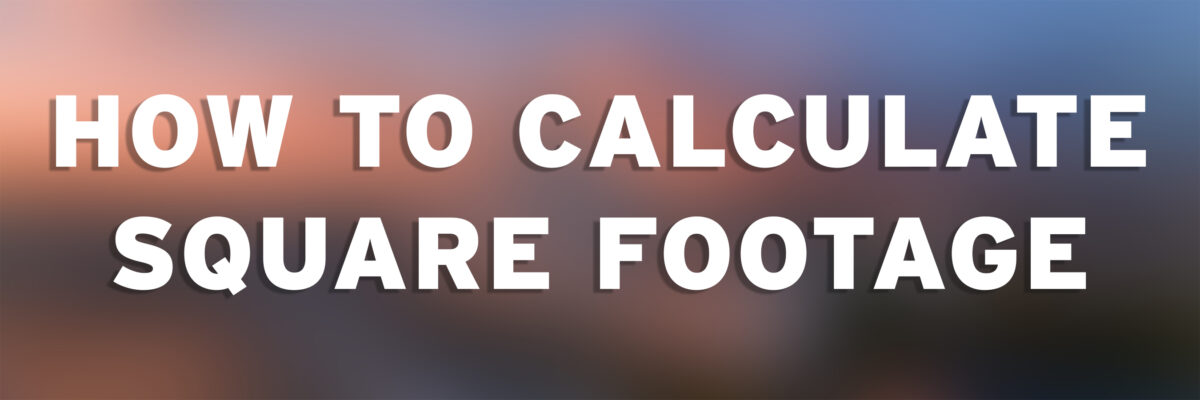# Math 101All of our inkjet printers are billed by the square foot. You are only charged for your image size and not the excess white paper. There are a few instances where the IC has sheets of inkjet media but the majority of all inkjet prints are printed on roll media.

This formula shows how to calculate the final price of your print job. All measurements must be in inches.

Length” × Width” = Square Inches”

Square Inches” / 144 = Square Feet’

Square Feet’ × Paper Cost = Cost of Print

For example, if you wanted to print an 27” x 38” image on the Epson 9900 on Enhanced Matte.

Step 1: 27” × 38” = 1026”

Step 2: 1026” / 144 = 7.125 sq. ft.

Step 3: 7.125 (round up to 7.13) sq. ft. × \$6.00 = \$42.78

The total cost for this print job would be \$42.78 before tax and \$45.35 after tax.

To figure out the price of your poster with tax, multiple the before-taxed amount by \$1.06.

## Calculator

Square Foot Calculator